# Statistics - math word problems

1. Float boya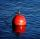A 0.5 meter spherical float is used as a location mark for a fishing boat anchor. It floats in salt water. Find the depth to which the float sinks if the material of which the float is made weighs 8 kilograms per cubic meter and salt water weighs 1027 kg/
2. Tributaries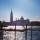The first tributary fill pool with water in 15 hours. The second tributary fill pool in 10 hours. For how many hours the pool is filled with both tributaries?
3. Forth and back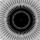Car goes from point A to point B at speed 78 km/h and back at 82 km/h. If went there and back at speed 81 km/h journey would take five minutes less. What is the distance between points A and B?
4. Boots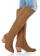Master shoemaker has three apprentices. First do one pair of boots for two days, second for 1 day, third on 1.5 day. If they worked together, for how long it would take made a couple of boots?
5. Inscribed circle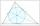Write the equation of a incircle of the triangle KLM if K [2,1], L [6,4], M [6,1].
6. The dam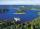The water reservoir is filled with first tributary for 25 hours second tributary for 35 hours. Some time were both inlets open, then a second tributary closed and the tank was filled in four hours. What time water flowed from both tributaries? (Expressed
7. Grass garden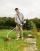Mr. Grass would itself be mowed garden for 5 hours. His son Michael could do it for 7 hours. They agreed that they wake up at 5 am and begins to mow together. Ms. Grass needs drive into town at 8.30. Michael says that at that time they're done with work.
8. Two workers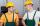Two workers work together and perform the job in 12 hours. If one has done half the work and then second worker next half of work it will take 25 hours. How long would it take job them separately?
9. Average mark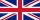Calculate average mark in English, if a student got a couple of 3's, 20% less 2 than 3, and 50% more 1's than the 2's.
10. Classs meanClass size is 30 students, math was not worse than sign 2. Determine the number of pupils who were sign 1 if the class had a 1.4 sign average.
11. Vinegar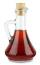What percentage vinegar we get if we mix 1 if dm³ eight percent vinegar with 1.5 dm³ six percent vinegar?
12. SD - meanMean is 10 and standard deviation is 3.5. If the data set contains 40 data values, approximately how many of the data values will fall within the range 6.5 to 13.5?
13. Annual incomeThe annual incomes (in thousands of \$) of fifteen families is: 60, 80, 90, 96, 120, 150, 200, 360, 480, 520, 1060, 1200, 1450, 2500, 7200 Calculate harmonic and geometric mean.
14. Box and whisker plotConstruct a box and whisker plot for the given data. 56, 32, 54, 32, 23, 67, 23, 45, 12, 32, 34, 24, 36, 47, 19, 43
15. AverageIf the average(arithmetic mean) of three numbers x,y,z is 50. What is the average of there numbers (3x +10), (3y +10), (3z+10) ?
16. Balls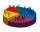We have n identical balls (numbered 1-n) is selected without replacement. Determine 1) The probability that at least one tensile strength number coincides with the number of balls? 2) Determine the mean and variance of the number of balls, which coincides
17. Triangle midpoints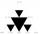Determine coordinates of triangle ABC vertices if we know tirangle sides midpoints SAB [0;3] SBC [1;6] SAC [4;5], its sides AB, BC, AC.
18. Arithmetic averageThe arithmetic mean of the five numbers is exactly 8. The sum of these four numbers is 30. What is the fifth number?
19. Enterprise and wages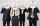The enterprise operates a total 162 workers and their average salary is M dollars. If the company has hire an additional 18 employees whose average wage would be the S dollars, would reduce the overall average salary in the company of 3.6%. Calculate th
20. Painters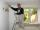The first team of painters would paint bridge in 15 days, the second in 10 days. After 3 days of working together second team goes out and continue only first team. How many days took second team to finish painting the bridge?

Do you have an interesting mathematical word problem that you can't solve it? Submit math problem, and we can try to solve it.

We will send a solution to your e-mail address. Solved examples are also published here. Please enter the e-mail correctly and check whether you don't have a full mailbox.

Please do not submit problems from current active competitions such as Mathematical Olympiad, correspondence seminars etc...